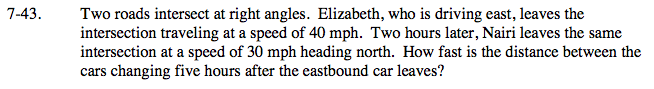### Home > CALC > Chapter 7 > Lesson 7.1.4 > Problem7-43

7-43.Let the eastbound car be at a distance x from the intersection and the northbound car at distance y.
Write an equation for the distance between them.

Implicitly differentiate the distance equation with respect to time. This will create a rate equation.

Evaluate.

≈ 48.788 mph